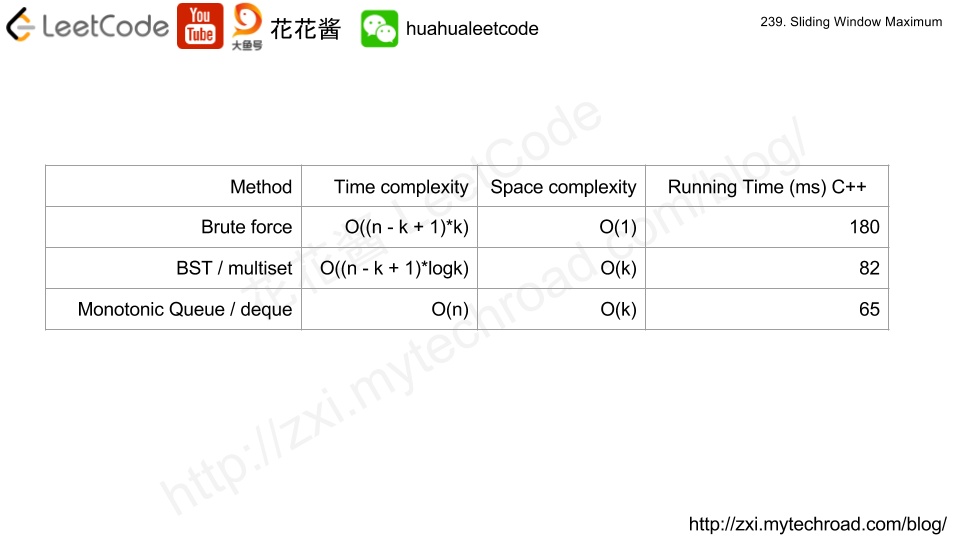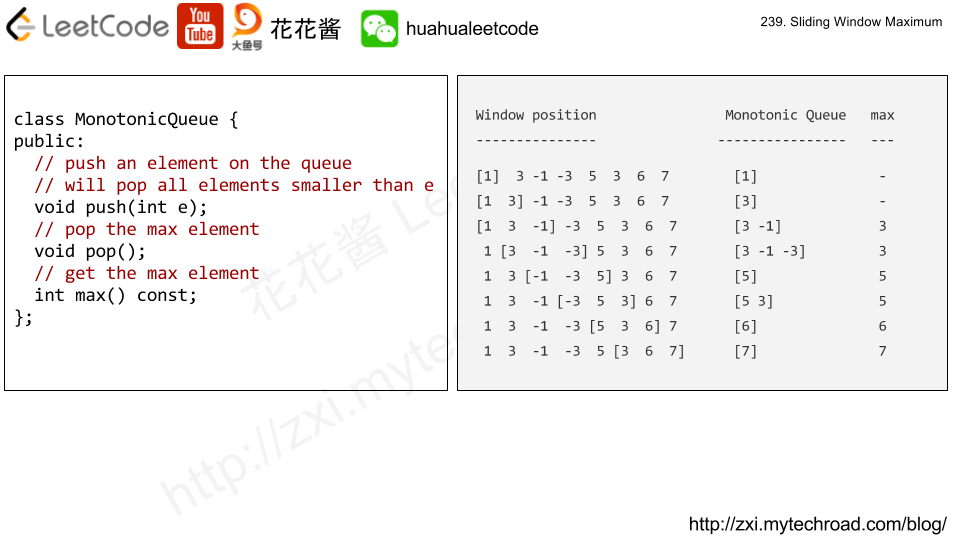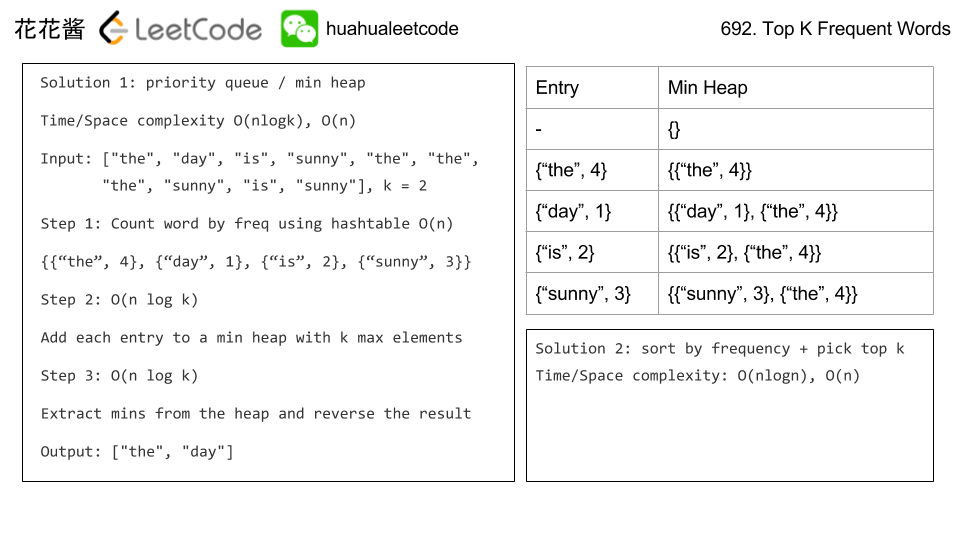# Posts published in “Heap”

You are driving a vehicle that has capacity empty seats initially available for passengers.  The vehicle only drives east (ie. it cannot turn around and drive west.)

Given a list of tripstrip[i] = [num_passengers, start_location, end_location] contains information about the i-th trip: the number of passengers that must be picked up, and the locations to pick them up and drop them off.  The locations are given as the number of kilometers due east from your vehicle’s initial location.

Return true if and only if it is possible to pick up and drop off all passengers for all the given trips.

Example 1:

Input: trips = [[2,1,5],[3,3,7]], capacity = 4
Output: false


Example 2:

Input: trips = [[2,1,5],[3,3,7]], capacity = 5
Output: true


Example 3:

Input: trips = [[2,1,5],[3,5,7]], capacity = 3
Output: true


Example 4:

Input: trips = [[3,2,7],[3,7,9],[8,3,9]], capacity = 11
Output: true

## Solution1: Min heap

Sort events by location

Time complexity: O(nlogn)
Space complexity: O(n)

## Solution 2: Preprocessing

Time complexity: O(n)
Space complexity: O(1000)

## C++

On an N x N grid, each square grid[i][j] represents the elevation at that point (i,j).

Now rain starts to fall. At time t, the depth of the water everywhere is t. You can swim from a square to another 4-directionally adjacent square if and only if the elevation of both squares individually are at most t. You can swim infinite distance in zero time. Of course, you must stay within the boundaries of the grid during your swim.

You start at the top left square (0, 0). What is the least time until you can reach the bottom right square (N-1, N-1)?

Example 1:

Input: [[0,2],[1,3]]
Output: 3
Explanation:
At time 0, you are in grid location (0, 0).
You cannot go anywhere else because 4-directionally adjacent neighbors have a higher elevation than t = 0.

You cannot reach point (1, 1) until time 3.
When the depth of water is 3, we can swim anywhere inside the grid.


Example 2:

Input: [[0,1,2,3,4],[24,23,22,21,5],[12,13,14,15,16],[11,17,18,19,20],[10,9,8,7,6]]
Output: 16
Explanation:
0  1  2  3  4
24 23 22 21  5
12 13 14 15 16
11 17 18 19 20
10  9  8  7  6

The final route is marked in bold.
We need to wait until time 16 so that (0, 0) and (4, 4) are connected.


Note:

1. 2 <= N <= 50.
2. grid[i][j] is a permutation of [0, …, N*N – 1].

## Solution 1: Dijkstra’s Algorithm

Time complexity: O(n^2*logn)
Space complexity: O(n^2)

## Solution 2: Binary Search + BFS

Time complexity: O(2logn * n^2)
Space complexity: O(n^2)

# Problem

Design a class to find the kth largest element in a stream. Note that it is the kth largest element in the sorted order, not the kth distinct element.

Your KthLargest class will have a constructor which accepts an integer k and an integer array nums, which contains initial elements from the stream. For each call to the method KthLargest.add, return the element representing the kth largest element in the stream.

Example:

int k = 3;
int[] arr = [4,5,8,2];
KthLargest kthLargest = new KthLargest(3, arr);


Note:
You may assume that nums‘ length ≥ k-1 and k ≥ 1.

# Solution: BST / Min Heap

Time complexity: O(nlogk)

Space complexity: O(k)

C++ / BST

C++ / Min Heap

Problem:

https://leetcode.com/problems/sliding-window-maximum/

Given an array nums, there is a sliding window of size k which is moving from the very left of the array to the very right. You can only see the k numbers in the window. Each time the sliding window moves right by one position.

For example,
Given nums = [1,3,-1,-3,5,3,6,7], and k = 3.

Therefore, return the max sliding window as [3,3,5,5,6,7].

Note:
You may assume k is always valid, ie: 1 ≤ k ≤ input array’s size for non-empty array.

Could you solve it in linear time?

Idea:# Solution 1: Brute Force

Time complexity: O((n – k + 1) * k)

Space complexity: O(1)

# Solution 2: BST

Time complexity: O((n – k + 1) * logk)

Space complexity: O(k)

# Solution 3: Monotonic Queue

Time complexity: O(n)

Space complexity: O(k)

## Python3 V2

Problem:

Given a non-empty list of words, return the k most frequent elements.

Your answer should be sorted by frequency from highest to lowest. If two words have the same frequency, then the word with the lower alphabetical order comes first.

Example 1:

Example 2:

Note:

1. You may assume k is always valid, 1 ≤ k ≤ number of unique elements.
2. Input words contain only lowercase letters.

1. Try to solve it in O(n log k) time and O(n) extra space.

Idea:

Priority queue / min heapSolution

C++ / priority_queue O(n log k) / O(n)

Related Problems

Mission News Theme by Compete Themes.